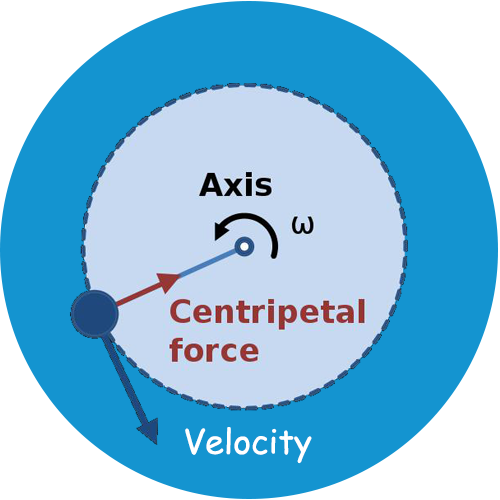# Centripetal Force Calculator

Use our Centripetal force calculator a free online tool to calculate the centripetal force of an object. Enter the values of velocity, mass and radius to calculate centripetal force.

When an object moves in a constant speed in a circular path, it is said to be moving in uniform circular motion. When an object moves in uniform circular motion there is a centripetal force acting on the object. A centripetal force is a force acting on an object, directed toward the center of the object’s circular path.

## Centripetal Force Formula

The product of the mass of the object and the centripetal acceleration is the centripetal force.

Fc = m ac = m v2 / r

where,
Fc = centripetal force in Newton = 1 kg * m/s2
m = the mass of the object moving in uniform circular motion (kg)
ac = centripetal acceleration (m/s2)